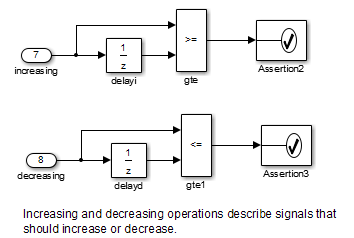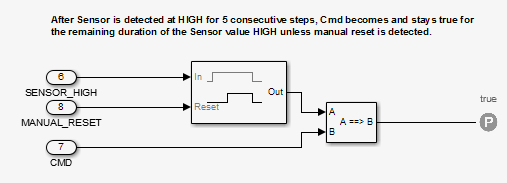## Model Requirements

The Simulink® Design Verifier™ block library includes a sublibrary Example Properties. The Example Properties sublibrary includes:

• Basic Properties — Four examples that demonstrate how to prove basic properties.

• Temporal Properties — Four examples that demonstrate how to define temporal properties on Boolean signals

The workflow for using these examples in your model is:

1. Copy these examples into your Verification Subsystem block.

2. Adapt them, if required, for the specific properties that you want to prove.

3. Run the Simulink Design Verifier analysis to prove that the assertions in these examples never fail.

4. If the assertion fails, the software creates a counterexample that causes the assertion to fail and then generates a harness model.

5. On the harness model, execute the counterexample to confirm that the assertion fails with that counterexample.

### Basic Properties

To view the Basic Properties examples:

1. Open the Simulink Design Verifier block library. Type:

`sldvlib`
2. Double-click the Examples sublibrary.

3. Double-click the Basic Properties block that contains the examples.

The sections that follow describe each example in the Block Properties sublibrary in detail.

#### Conditions that Trigger a Result

The Simulink Design Verifier Implies block allows you to test for conditions that trigger a result. This example specifies that if condition `A` is true, result `B` must always be true.#### Increasing or Decreasing Signals

The two examples in this section specify that a signal is either:

• Always increasing or staying constant

• Always decreasing or staying constant#### Exclusivity Operation

This example describes four conditions that should not be true at the same time.#### Conditions with One True Element

This example specifies that only one of the four input signals can be true.### Temporal Properties

To view the Temporal Properties examples:

1. Open the Simulink Design Verifier block library. Type:

`sldvlib`
2. Double-click the Temporal Properties sublibrary.

3. Double-click the Temporal Properties block that contains the examples.

The sections that follow describe each example in the Temporal Properties sublibrary in detail.

#### Synchronize the Output with the Input

When the input `In1` equals `ACTIVE`, the input `In2` is set to `INACTIVE` after five time steps.#### Make a Signal Inactive After a Delay

In this example, after five consecutive time steps where the `SENSOR_HIGH` input is true, the `CMD` signal becomes true. `CMD` is true as long as `SENSOR_HIGH` is true, unless the block is reset by the `MANUAL_RESET` signal.#### Extend a True Signal

In this example, after the input becomes true, the output becomes true for the number of time steps specified in the Detector block, in this case, 5. The input remains true for 5 time steps as well.#### Test the Input Against a Specified Threshold

When the input `In3` equals `ON` and the input `In4` is less than the constant `THRESHOLD`, `In3` is set to `OFF` within five time steps.